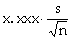## Two Technical Details

(1) Simple random sampling is usually done without replacement (so there is no chance of the same individual appearing twice in the sample). In this case, there is a bit less exposure to sampling error than in sampling with replacement.

 the standard error of the mean (when sampling without replacement from a population of size N)In most managerial applications, the population size is much larger than the sample size; frequently the population size isn't even known. As long as N is much larger than n, the final factor in the equation above is very close to 1. And in all cases, it's no more than 1. Therefore, omitting it from our calculations, and treating sampling with and without replacement as being the same, is completely benign, and is usually done in practice.

(2) If the sample size is much below 20, the assumption that the sample mean is approximately normally distributed is questionable. In general, a 95%-confidence interval for the estimate cannot be given: The estimate should just be reported along with a warning that the sample size was quite small. (The actual sample data can be attached to the warning, since it will fill less than a single page.)

However, if the population itself is approximately normally distributed, then a 95%-confidence interval for the estimate can be reported. Still, in both this special small-sample case and in the general larger-sample case, we're cheating a bit when we use s instead of σ. Taking this into account, we need to make a slight adjustment in the margin-of-error formula:

 the margin of error of the estimate (at the 95%-confidence level), where x.xxx comes from the t-distribution with n-1 degrees of freedom (see the table below)t-distribution
degrees of freedom 95% central probability degrees of freedom 95% central probability degrees of freedom 95% central probability
1 12.706 11 2.201 21 2.080
2 4.303 12 2.179 22 2.074
3 3.182 13 2.160 23 2.069
4 2.776 14 2.145 24 2.064
5 2.571 15 2.131 25 2.060
6 2.447 16 2.120 30 2.042
7 2.365 17 2.110 40 2.021
8 2.306 18 2.101 60 2.000
9 2.262 19 2.093 120 1.980
10 2.228 20 2.086 1.960

Note that, as the sample size grows, the correct “approximately 2” multiplier becomes closer and closer to 1.96.